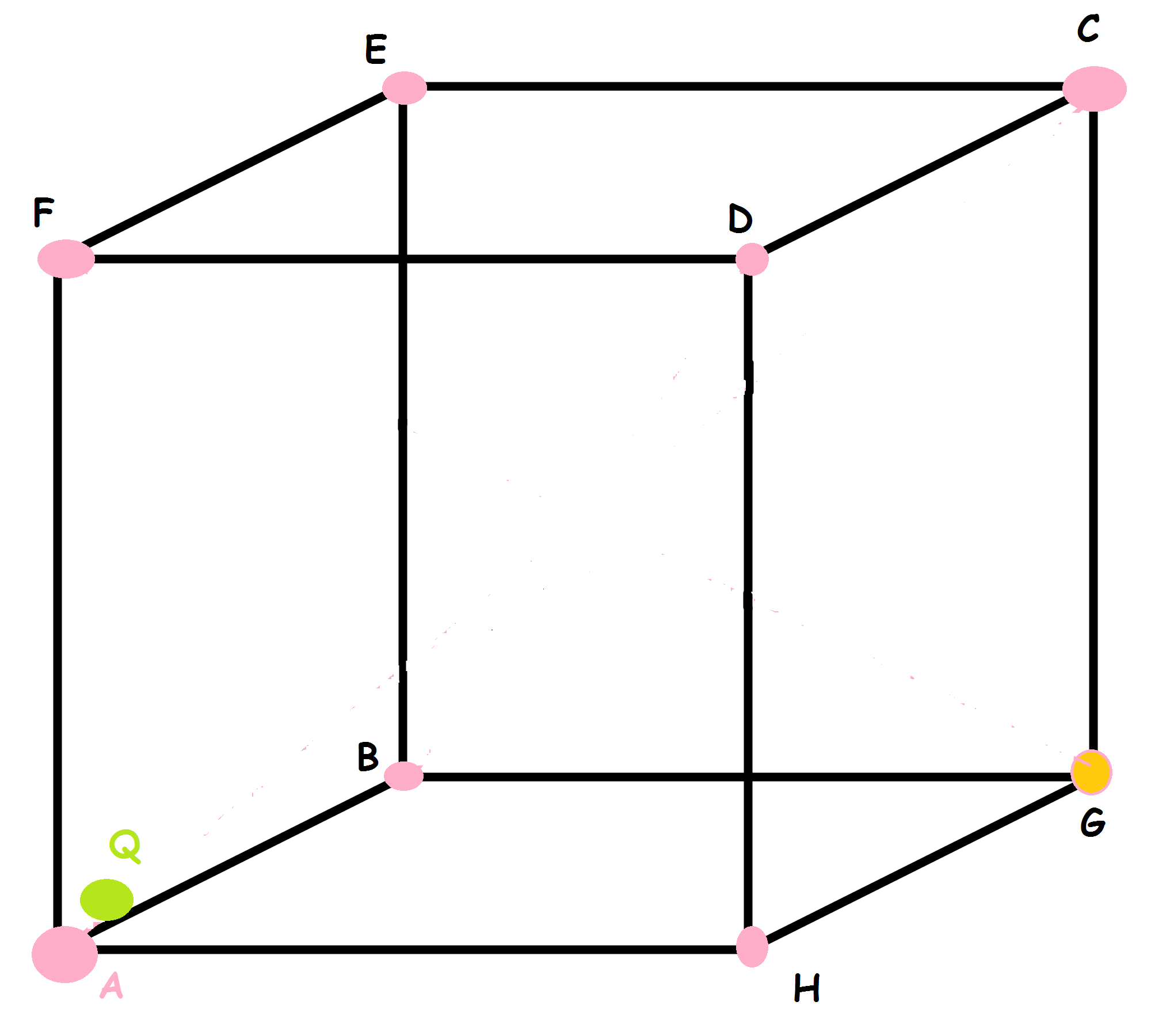# Cube! Not related to geometry this time

A point Charge Q is placed just inside the cube AHDFECGB near the vertex A . Find the total flux through the faces AHDF, AFEB and AHGB.

The Answer is of form $\dfrac{kQ}{x\epsilon}$. Here k and x are co-prime integers. Find the value of $\boxed{k+x}$.

Hint: For those having problems in visualising the cube, AHDF is the face towards you and AHGB is the base of cube.This Problem is Original.

×

Problem Loading...

Note Loading...

Set Loading...